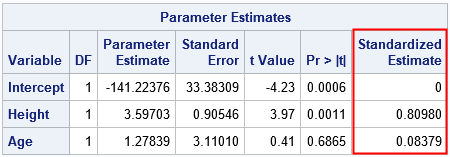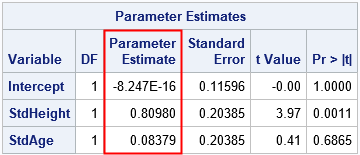A SAS programmer recently asked how to interpret the "standardized regression coefficients" as computed by the STB option on the MODEL statement in PROC REG and other SAS regression procedures. The SAS documentation for the STB option states, "a standardized regression coefficient is computed by dividing a parameter estimate by the ratio of the sample standard deviation of the dependent variable to the sample standard deviation of the regressor." Although correct, this definition does not provide an intuitive feeling for how to interpret the standardized regression estimates. This article uses SAS to demonstrate how parameter estimates for the original variables are related to parameter estimates for standardized variables. It also derives how regression coefficients change after a linear transformation of the variables.

### Proof by example

One of my college physics professors used to smile and say "I will now prove this by example" when he wanted to demonstrate a fact without proving it mathematically. This section uses PROC STDIZE and PROC REG to "prove by example" that the standardized regression estimates for data are equal to the estimates that you obtain by standardizing the data. The following example uses continuous response and explanatory variables, but there is a SAS Usage Note that describes how to standardize classification variables.

The following call to PROC REG uses the STB option to compute the standardized parameter estimates for a model that predicts the weights of 19 students from heights and ages:

```proc reg data=Sashelp.Class plots=none; Orig: model Weight = Height Age / stb; ods select ParameterEstimates; quit;```The last column is the result of the STB option on the MODEL statement. You can get the same numbers by first standardizing the data and then performing a regression on the standardized variables, as follows:

```/* Put original and standardized variables into the output data set. Standardized variables have the names 'StdX' where X was the name of the original variable. METHOD=STD standardizes variables according to StdX = (X - mean(X)) / std(X) */ proc stdize data=Sashelp.Class out=ClassStd method=std OPREFIX SPREFIX=Std; run;   proc reg data=ClassStd plots=none; Std: model StdWeight = StdHeight StdAge; ods select ParameterEstimates; quit;```The parameter estimates for the standardized data are equal to the STB estimates for the original data. Furthermore, the t values and p-values for the slope parameters are equivalent because these statistics are scale- and translation-invariant. Notice, however, that scale-dependent statistics such as standard errors and covariance of the betas will not be the same for the two analyses.

### Linear transformations of random variables

Mathematically, you can use a algebra to understand how linear transformations affect the relationship between two linearly dependent random variables. Suppose X is a random variable and Y = b0 + b1*X for some constants b0 and b1. What happens to this linear relationship if you apply linear transformations to X and Y?

Define new variables U = (Y - cY) / sY and V = (X - cX) / sX. If you solve for X and Y and plug the expressions into the equation for the linear relationship, you find that the new random variables are related by
U = (b0 + b1*cX - cY) / sY + b1*(sX/sY)*V.
If you define B0 = (b0 + b1*cX - cY) / sY and B1 = b1*(sX/sY), then U = B0 + B1*V, which shows that the transformed variables (U and V) are linearly related.

The physical significance of this result is that linear relationships persist no matter what units you choose to measure the variables. For example, if X is measured in inches and Y is measured in pounds, then the quantities remain linearly related if you measure X in centimeters and measure Y in "kilograms from 20 kg."

### The effect of standardizing variables on regression estimates

The analysis in the previous section holds for any linear transformation of linearly related random variables. But suppose, in addition, that

• U and V are standardized versions of Y and X, respectively. That is, cY and cX are the sample means and sY and sX are the sample standard deviations.
• The parameters b0 and b1 are the regression estimates for a simple linear regression model.

For simple linear regression, the intercept estimate is b0 = cY - b1*cY, which implies that B0 = 0. Furthermore, the coefficient B1 = b1*(sX/sY) is the original parameter estimate "divided by the ratio of the sample standard deviation of the dependent variable to the sample standard deviation of the regressor," just as the PROC REG documentation states and just as we saw in the PROC REG output in the previous section. Thus the STB option on the MODEL statement does not need to standardize any data! It produces the standardized estimates by setting the intercept term to 0 and dividing the parameter estimates by the ratio of standard deviations, as noted in the documentation. (A similar proof handles multiple explanatory variables.)

### Interpretation of the regression coefficients

For the original (unstandardized) data, the intercept estimate predicts the value of the response when the explanatory variables are all zero. The regression coefficients predict the change in the response for one unit change in an explanatory variable. The "change in response" depends on the units for the data, such as kilograms per centimeter.

The standardized coefficients predict the number of standard deviations that the response will change for one STANDARD DEVIATION of change in an explanatory variable. The "change in response" is a unitless quantity. The fact that the standardized intercept is 0 indicates that the predicted value of the (centered) response is 0 when the model is evaluated at the mean values of the explanatory variables.

In summary, standardized coefficients are the parameter estimates that you would obtain if you standardize the response and explanatory variables by centering and scaling the data. A standardized parameter estimate predicts the change in the response variable (in standard deviations) for one standard deviation of change in the explanatory variable.

The post Standardized regression coefficients appeared first on The DO Loop.